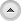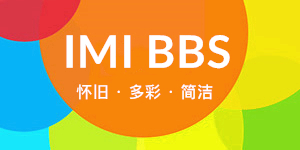##function text() { }; text = new text(); number = 0; text[number++] = "IMI BBS，一款怀旧小米社区的风格！" text[number++] = "每天一点正能量：复杂永远拼不过简单." text[number++] = "面对失败和挫折，一笑而过是一种乐观自信。" text[number++] = "小米风格社区模板已上架应用中心，欢迎前往选购。" text[number++] = "海誓山盟无需太多，陪伴，就是最好的承诺。" text[number++] = "学习从来无捷径，循序渐进登高峰。" text[number++] = "春暖花开，执子之手，人间值得" text[number++] = "追梦之路，阳光常伴，偶有风雨，心无阻，脚不停。" text[number++] = "永远的繁华在于不断的创新，创新即新契机。" text[number++] = "人生天地之间，若白驹之过隙，忽然而已。" text[number++] = "是否，你一直在想自己要成为一个什么样的人？" text[number++] = "这里的内容可以自定义，每次刷新都会显示不同内容" increment = Math.floor(Math.random() * number); document.write(text[increment]);## 关于我们